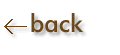Scientific Notation:  Powers of Ten
1. Rules for writing numbers in scientific notation:
2. Write all significant figures but only the significant figures.
3. Place the decimal point after the first digit, making the number have a value between 1 and 10.
4. Use the correct power of ten to place the decimal point properly, as indicated below.

a)  Positive exponents push the decimal point to the right.  The number becomes larger.

It is multiplied by the power of 10.

b)  Negative exponents push the decimal point to the left.  The number becomes smaller.

It is divided by the power of 10.

c)  10o  =  1

Examples:    3400  =  3.20 x 103                0.0120  =  1.20 x 10-2

Nice visual display of Powers of Ten (a view from outer space to the inside of an atom) viewed by powers of 10!